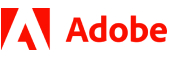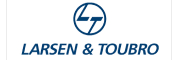New update is available. Click here to update.
Topics

# Change Start Node

Easy0/40
Average time to solve is 20m+3 more companies

## Problem statement

You are given a singly linked list and an integer ‘K’. You are supposed to make ‘K’ th node from the end of a linked list as the starting node of the linked list.

Note :
``````Given ‘K’ will always be valid.
``````
Detailed explanation ( Input/output format, Notes, Images )
Constraints :
``````1 <= N  <= 5*10^5
1 <= K <= N
-10^9 <= data <= 10^9 and data != -1

Time Limit : 1 sec
``````
Sample Input 1:
``````7 11 13 17 19 23 29 -1
3
``````
Sample Output 1:
``````19 7 11 13 17 23 29 -1
``````
Explanation For Sample Input 1:
``````Removing the third node from the end of the linked list, i.e. 19 and make the head node of the linked list.
``````
Sample Input 2:
``````13 1 19 3 9 -1
5
``````
Sample Output 2:
``````13 1 19 3 9 -1
``````Console